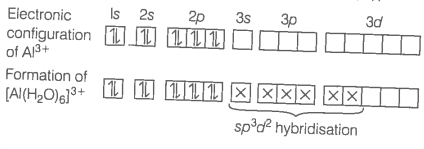When $BC{l}_{3}$ is treated with water, it hydrolysis and forms $\left[B{\left(OH\right)}_{4}{\right]}^{-}$ on whereas $AlC{l}_{3}$ in acidified aqueous solution forms $\left[Al{\left({H}_{2}O\right)}_{6}{\right]}^{3+}$ ion. Explain what is the hybridisation or boron and aluminium in these species ?

In trivalent state, most of the compounds being covalent are hydrolysed in water, e.g., BC on hydrolysis in water form ${\left[\mathrm{B}{\left(\mathrm{OH}\right)}_{4}\right]}^{-}$ species, the hybridisation state of B is sp3.
$\mathrm{BCl}+3{\mathrm{H}}_{2}\mathrm{O}\to \mathrm{B}{\left(\mathrm{OH}\right)}_{3}+3\mathrm{HCl}\phantom{\rule{0ex}{0ex}}\mathrm{B}{\left(\mathrm{OH}\right)}_{3}+{\mathrm{H}}_{2}\mathrm{O}\to {\left[\mathrm{B}{\left(\mathrm{OH}\right)}_{4}\right]}^{-}+{\mathrm{H}}^{+}$
AlCl3 in acidified aqueous solution form octahedral ${\left[\mathrm{Al}{\left({\mathrm{H}}_{2}\mathrm{O}\right)}_{6}\right]}^{3+}$ ion. In this complex, the 3d orbitals of Al are involved and the hybridisation state of Al is sp3d2.
${\mathrm{AlCl}}_{3}+{\mathrm{H}}_{2}\mathrm{O}\stackrel{\mathrm{HCl}}{\to }{\left[\mathrm{Al}{\left({\mathrm{H}}_{2}\mathrm{O}\right)}_{6}\right]}^{3+}+3{\mathrm{Cl}}^{-}\left(\mathrm{aq}\right)$